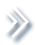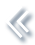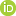####ArchivesReview Articles

## Optimal model averaging estimator for multinomial logit models

Rongjie Jiang ,

School of Statistics and Management, Shanghai University of Finance and Economics, Shanghai, People's Republic of ChinaLiuming Wang ,

School of Statistics and Management, Shanghai University of Finance and Economics, Shanghai, People's Republic of ChinaYang Bai

School of Statistics and Management, Shanghai University of Finance and Economics, Shanghai, People's Republic of Chinastatbyang@mail.shufe.edu.cn

Pages | Received 29 Mar. 2020, Accepted 10 Jan. 2022, Published online: 17 Feb. 2022,
• Abstract
• References
• Citations

In this paper, we study optimal model averaging estimators of regression coefficients in a multinomial logit model, which is commonly used in many scientific fields. A Kullback–Leibler (KL) loss-based weight choice criterion is developed to determine averaging weights. Under some regularity conditions, we prove that the resulting model averaging estimators are asymptotically optimal. When the true model is one of the candidate models, the averaged estimators are consistent. Simulation studies suggest the superiority of the proposed method over commonly used model selection criterions, model averaging methods, as well as some other related methods in terms of the KL loss and mean squared forecast error. Finally, the website phishing data is used to illustrate the proposed method.

## References

• Abdelhamid, N., Ayesh, A., & Thabtah, F. (2014). Phishing detection based associative classification data mining. Expert Systems with Applications41(13), 5948–5959. https://doi.org/10.1016/j.eswa.2014.03.019
• Akaike, H. (1973). Maximum likelihood identification of Gaussian autoregressive moving average models. Biometrika60(2), 255–265. https://doi.org/10.1093/biomet/60.2.255
• Ando, T., & Li, K. C. (2014). A model-averaging approach for high-dimensional regression. Journal of the American Statistical Association109, 254–265. https://doi.org/10.1080/01621459.2013.838168
• Bayaga, A. (2010). Multinomial logistic regression: Usage and application in risk analysis. Journal of Applied Quantitative Methods5, 288–297.
• Buckland, S. T., Burnham, K. P., & Augustin, N. H. (1997). Model selection: An integral part of inference. Biometrics53(2), 603–618. https://doi.org/10.2307/2533961
• Cavanaugh, J. E. (1999). A large-sample model selection criterion based on Kullback's symmetric divergence. Statistics & Probability Letters42(4), 333–343. https://doi.org/10.1016/S0167-7152(98)00200-4
• Cheng, T. C. F., Ing, C. K., & Yu, S. H. (2015). Toward optimal model averaging in regression models with time series errors. Journal of Econometrics189(2), 321–334. https://doi.org/10.1016/j.jeconom.2015.03.026
• Diebold, F. X., & Mariano, R. S. (2002). Comparing predictive accuracy. Journal of Business & Economic Statistics20, 134–144. https://doi.org/10.1198/073500102753410444
• Ederington, L. H. (1985). Classification models and bond ratings. Financial Review20, 237–262. https://doi.org/10.1111/fire.1985.20.issue-4
• Fahrmeir, L., & Tutz, G. (2013). Multivariate statistical modelling based on generalized linear models. Springer Science & Business Media.
• Friedman, J., Hastie, T., & Tibshirani, R. (2010). Regularization paths for generalized linear models via coordinate descent. Journal of Statistical Software33, 1–22. https://doi.org/10.18637/jss.v033.i01
• Guadagni, P. M., & Little, J. D. C. (1983). A logit model of brand choice calibrated on scanner data. Marketing Science2, 203–238. https://doi.org/10.1287/mksc.2.3.203
• Hansen, B. E. (2007). Least squares model averaging. Econometrica75, 1175–1189. https://doi.org/10.1111/ecta.2007.75.issue-4
• Hansen, B. E., & Racine, J. S. (2012). Jackknife model averaging. Journal of Econometrics167, 38–46. https://doi.org/10.1016/j.jeconom.2011.06.019
• Hoeting, J. A., Madigan, D., Raftery, A. E., & Volinsky, C. T. (1999). Bayesian model averaging: A tutorial. Statistical Science14, 382–417. https://doi.org/10.1214/ss/1009212519
• Hurvich, C. M., Simonoff, J. S., & Tsai, C. L. (1998). Smoothing parameter selection in nonparametric regression using an improved Akaike information criterion. Journal of the Royal Statistical Society: Series B (Statistical Methodology)60, 271–293. https://doi.org/10.1111/1467-9868.00125
• Konishi, S., & Kitagawa, G. (1996). Generalised information criteria in model selection. Biometrika83, 875–890. https://doi.org/10.1093/biomet/83.4.875
• Li, C., Li, Q., Racine, J., & Zhang, D. Q. (2018). Optimal model averaging of varying coefficient models. Statistica Sinica28, 2795–2809. https://doi.org/10.5705/ss.202017.0034
• Liu, Q., & Okui, R. (2013). Heteroskedasticity-Robust CpCp model averaging. Econometrics Journal16(3), 463–472. https://doi.org/10.1111/ectj.12009
• Lu, X., & Su, L. (2015). Jackknife model averaging for quantile regressions. Journal of Econometrics188, 40–58. https://doi.org/10.1016/j.jeconom.2014.11.005
• Mallows, C. L. (1973). Some comments on Cpp. Technometrics15, 661–675. https://doi.org/10.1080/00401706.1973.10489103
• Portnoy, S. (1988). Asymptotic behavior of likelihood methods for exponential families when the number of parameters tends to infinity. The Annals of Statistics16(1), 356–366. https://doi.org/10.1214/aos/1176350710
• Raftery, A. E., & Zheng, Y. (2003). Discussion: Performance of Bayesian model averaging. Journal of the American Statistical Association98(464), 931–938. https://doi.org/10.1198/016214503000000891
• Schwarz, G. (1978). Estimating the dimension of a model. The Annals of Statistics6(2), 461–464. https://doi.org/10.1214/aos/1176344136
• Shen, X., Huang, H. C., & Ye, J. (2004). Adaptive model selection and assessment for exponential family distributions. Technometrics46(3), 306–317. https://doi.org/10.1198/004017004000000338
• Wan, A. T., Zhang, X., & Wang, S. (2014). Frequentist model averaging for multinomial and ordered logit models. International Journal of Forecasting30(1), 118–128. https://doi.org/10.1016/j.ijforecast.2013.07.013
• Wan, A. T., Zhang, X., & Zou, G. (2010). Least squares model averaging by Mallows criterion. Journal of Econometrics156(2), 277–283. https://doi.org/10.1016/j.jeconom.2009.10.030
• Wang, H., Zhang, X., & Zou, G. (2009). Frequentist model averaging estimation: A review. Journal of Systems Science and Complexity22(4), 732–748. https://doi.org/10.1007/s11424-009-9198-y
• White, H. (1982). Maximum likelihood estimation of misspecified models. Econometrica50(1), 1–25. https://doi.org/10.2307/1912526
• Zhang, X., & Liu, C. A. (2019). Inference after model averaging in linear regression models. Econometric Theory35(4), 816–841. https://doi.org/10.1017/S0266466618000269
• Zhang, X., Wan, A. T., & Zou, G. (2013). Model averaging by jackknife criterion in models with dependent data. Journal of Econometrics174(2), 82–94. https://doi.org/10.1016/j.jeconom.2013.01.004
• Zhang, X., & Wang, W. (2019). Optimal model averaging estimation for partially linear models. Statistica Sinica29, 693–718. https://doi.org/10.5705/ss.202015.0392
• Zhang, X., Yu, D., Zou, G., & Liang, H. (2016). Optimal model averaging estimation for generalized linear models and generalized linear mixed-effects models. Journal of the American Statistical Association111(516), 1775–1790. https://doi.org/10.1080/01621459.2015.1115762
• Zhang, X., & Yu, J. (2018). Spatial weights matrix selection and model averaging for spatial autoregressive models. Journal of Econometrics203(1), 1–18. https://doi.org/10.1016/j.jeconom.2017.05.021
• Zhang, X., Zou, G., & Carroll, R. J. (2015). Model averaging based on Kullback-Leibler distance. Statistica Sinica25, 1583–1598. https://doi.org/10.5705/ss.2013.326
• Zhang, X., Zou, G., Liang, H., & Carroll, R. J. (2020). Parsimonious model averaging with a diverging number of parameters. Journal of the American Statistical Association115(530), 972–984. https://doi.org/10.1080/01621459.2019.1604363
• Zhao, P., & Li, Z. (2008). Central limit theorem for weighted sum of multivariate random vector sequences. Journal of Mathematics28, 171–176. https://doi.org/10.1007/s12033-007-0073-6
• Zhao, S., Zhou, J., & Yang, G. (2019). Averaging estimators for discrete choice by M-fold cross-validation. Economics Letters174, 65–69. https://doi.org/10.1016/j.econlet.2018.10.014
• Zhu, R., Wan, A. T., Zhang, X., & Zou, G. (2019). A mallows-type model averaging estimator for the varying-coefficient partially linear model. Journal of the American Statistical Association114(526), 882–892. https://doi.org/10.1080/01621459.2018.1456936
• Zhu, R., Zou, G., & Zhang, X. (2018). Model averaging for multivariate multiple regression models. Statistics52(1), 205–227. https://doi.org/10.1080/02331888.2017.1367794

To cite this article: Rongjie Jiang, Liming Wang & Yang Bai (2022): Optimal model averaging estimator for multinomial logit models, Statistical Theory and Related Fields, DOI: 10.1080/24754269.2022.2037204